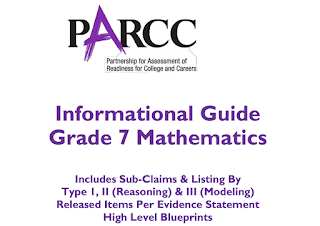## Labels

### Math 7 PARCC Reviewer 4 (7.RP.2c)

Sub-claim:
The students solve problems involving the Major Content with connections to the Standards for Mathematical Practice
Type of items: Type 1
Color Code: Peach
Evidence Statement Key: 7.RP.2c
Calculator: No
Point: 1 each (1:29)
Evidence Statement Text: Recognize and represent proportional relationships between quantities.
c. Represent proportional relationships by equations. For example, if total cost t is proportional to the number n of items purchased at a constant price p, the relationship between the total cost and the number of items can be expresses as t = pn.
Clarifications, limits, emphases, and other information intended to ensure appropriate variety in tasks:
Tasks use only coordinates in Quadrant 1 and use only a positive constant of proportionality.
Sample Questions (taken from PARCC’s Practice Tests and Released Items):
EOY Released Test Item 7
PBA Released Test Item 2
Practice Test, Unit1, Item 14
2016 Released Item #141. EOY Released Test Item 7
The amount Troy charges to mow a lawn is proportional to the time it takes him to mow the lawn. Troy charges \$30 to mow a lawn that took him 1.5 hours to mow. Which equation models the amount in dollar, d, Troy charges when it takes him h hours to mow a lawn?
a. d = 20h
b. h = 20d
c. d = 45h
d. h = 45d

2. PBA Released Test Item 2
The relationship between the number of ounces of tea purchased and the total cost of the tea is proportional, as shown in this graph.
Which equation models this relationship?
3. Practice Test, Unit 1, Item 14
Hayden mixed 6 cups of blue paint with 8 cups of yellow paint to make green paint. To represent the relationship between the number of cups of blue paint, b, and the number of cups of yellow paint, y, needed to make the same shade of green paint, Hayden wrote the equation b = ___y. What number should be placed on the blank? Enter your answer on the blank.

4. 2016 Released Item #14
An earthquake wave travels through 55 km of rock in 25 seconds. Which equation represents the relationship between t, the number of seconds, and d, the total distance the wave travels?